# “The First, Last and Only Superior Calculator in the World”

This general-purpose calculator for simple and complex calculations uses the entire screen to display its content. You can click on old results to select, instead of re-typing them.
It supports fractions, repetitive parts, BIG numbers, and all these options combined. If the calculation gets lengthy, you will always receive feedback on the calculation process.

Normal & Scientific Calculations, Fractions, Repetitive Parts, BIG Numbers

You can save the data on your screen for later use, export it or copy to the clipboard as text or as image, you can opt for just one line or the whole screen.

Examples:

If you copy the data on the screen above as text to the clipboard, you will get:

1 + 1 = 2

2 ^ 3 = 8

8 ^ 8 = 16777216

1 / 3 = 0, = 1/3

1/3 ^ 2 x 0, = 0, = 2/27

100 ! = 2 ^ 97 x 3 ^ 48 x 5 ^ 24 x 7 ^ 16 x 11 ^ 9 x 13 ^ 7 x 17 ^ 5 x 19 ^ 5 x 23 ^ 4 x 29 ^ 3 x 31 ^ 3 x 37 ^ 2 x 41 ^ 2 x 43 ^ 2 x 47 ^ 2 x 53 x 59 x 61 x 67 x 71 x 73 x 79 x 83 x 89 x 97

16777216

## Fractions

Fractions can be manipulated with the 4 buttons below: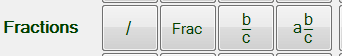Fraction division: the result will be displayed as a fraction.

Fraction division: the result will be displayed as a fraction.

## Repetitive parts

Repetitive parts are the repeating pattern of a number, like the result of 1 / 3:

... (goes on forever!)

Division goes on until the repetitive part is found, no matter how many decimals you work , with

Converts last result (a fraction) to a decimal with repetitive parts.

## Fractions and Repetitive parts combined

You can easily combine fractions and repetitive parts.

For example, if you type: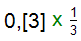You can use

- to get the result as a fraction,

and you can use

- to get the result as a repetitive part.

## 5 types of divisions

There are 5 types of divisions:

Decimal division: no repetitive parts, goes on until finished or until the maximum number of decimals is reached.

Repetitive part division: goes on until finished or until the repetitive part is found.

Fraction division: the result is displayed as a fraction.

Remainder division: the result is displayed as a whole number + remainder, like 2 / 3 = 0 REM 2.

Plus the default division: mixed-mode

Mixed-mode division: goes on until finished or until the maximum number of decimals is reached.
If a repetitive part is found before the maximum number of decimals is reached, then a repetitive part is given.

## Factorial" and "Factorization" + calculations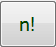A Factorial can be written as n!, where n is any whole number >= 0.

For example 10! = 10 x 9 x 8 x 7 x 6 x 5 x 4 x 3 x 2 x 1

Factorization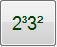The big fun comes in when you factorize a factorial.

Suppose you want to factorize the factorial of 1000.

This is what you'll get: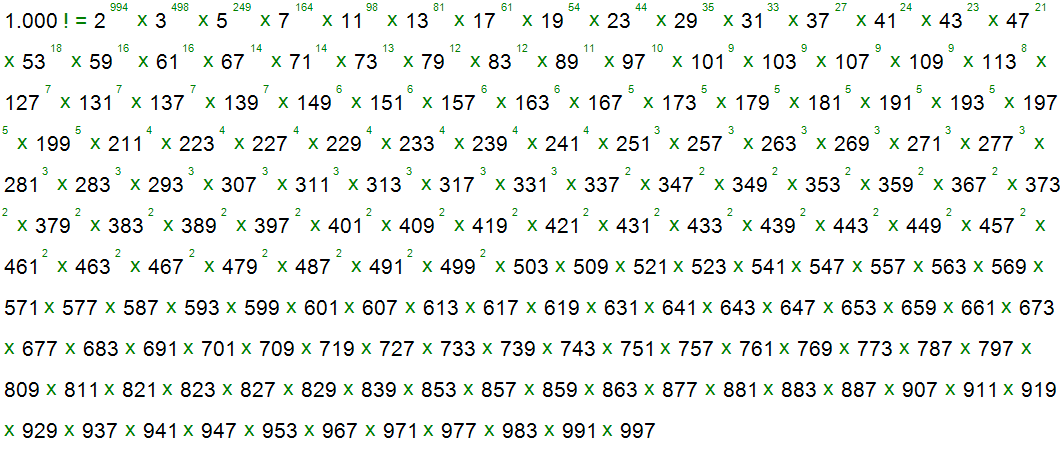## BIG Numbers

Do you want to have even more fun? Then start with BIG numbers. Not all calculations in this application can work with BIG numbers.

This is the most expanded version of the input panel:

The buttons for BIG fun with... and BIG Numbers support the big numbers.

The small fun section with functions like sine and cosine and the powers containing decimal numbers only give small fun with small results. This is like working with a normal calculator - you will get the same number of digits.

How BIG is BIG? The program crashes when the consumed memory is about 1 Gb. It will take a couple of hours until this condition is reached, depending on the type of calculations you're working with.

Decimals to a maximum of 10.000.000 digits

Enter the maximum number of decimals:

## BIG Fractions

We have BIG numbers and we have fractions. But what about BIG fractions? Well, we can assure you that this calculator has BIG fractions too! Suppose you want to create a fraction with the result of 9999! as nominator and the result of 10000! as denominator, like:

Can you do that ? Yes you can!

## How to view or edit your Biggies

Once you've created a BIG number or fraction, you can view it by clicking on the edit button. You'll find this button on the right side of the number:

You will get a screen like this: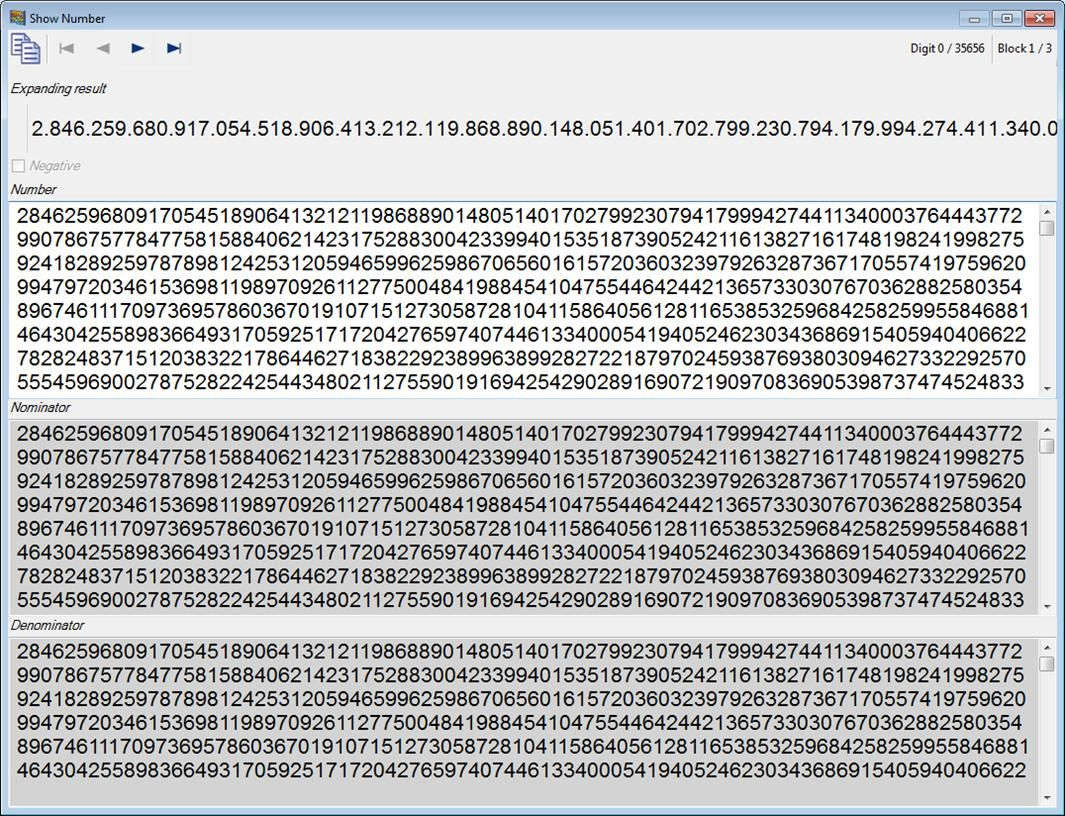If you want to edit the last calculated BIG number, like in the previous example: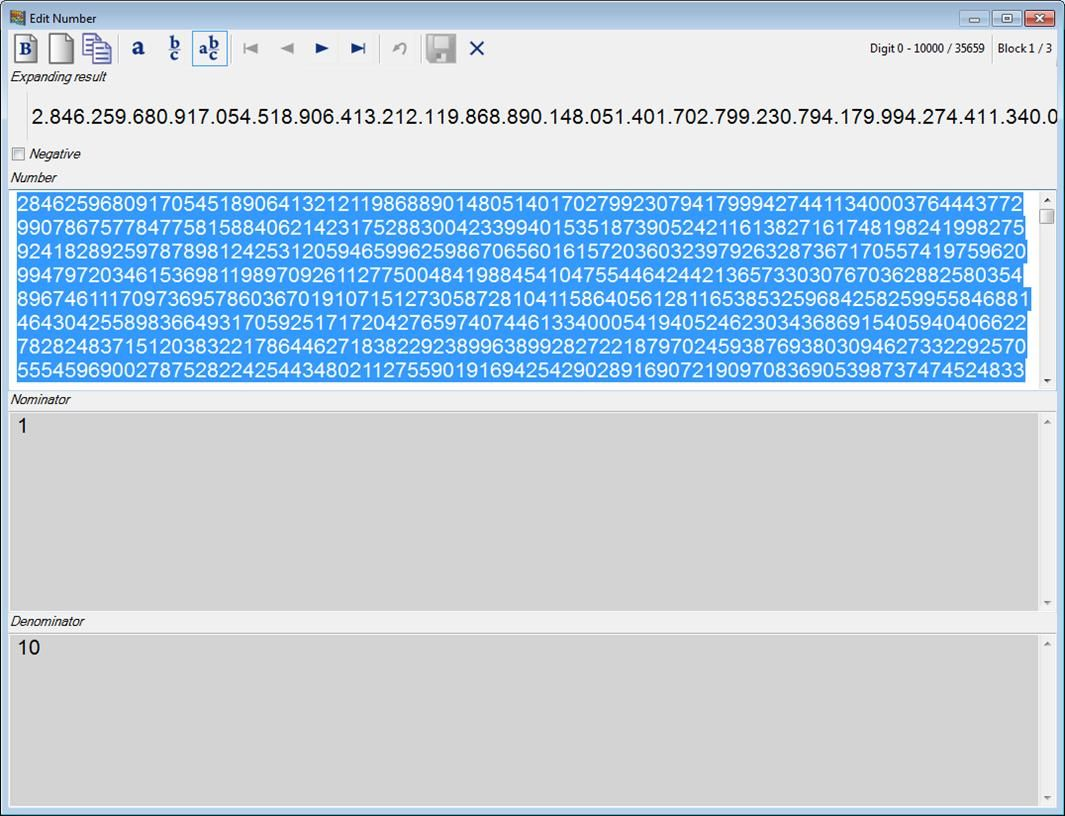Here you can view or edit the number, remove the fraction part, etc.

## You can always get feedback on lengthy calculations

Suppose you want to get your hands really dirty with a nice lengthy calculation, like:

You will always get feedback via the Calculation Progress Window: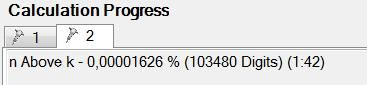You'll find the Calculation Progress Window on the right side of the button panel.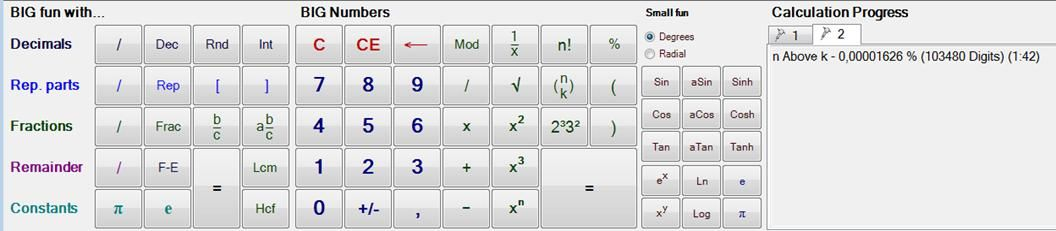## How to use the Heavy Duty Calculator as a Tool Window

In some situations you just need a small always-on-top window with no buttons to perform your calculations on, while having another document open.

Something like this:

To get a window like this, go to Options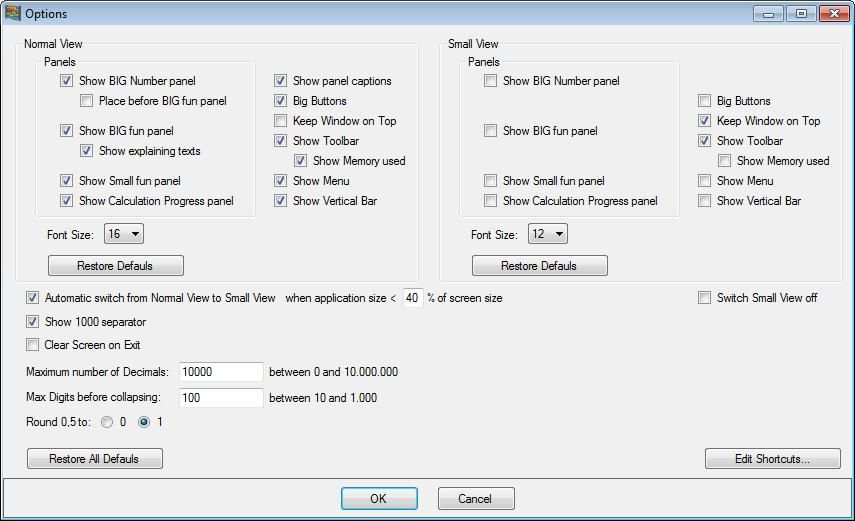Within Options, you are able to create two types of Views: Normal View and Small View. The application can switch automatically from one view to the other, depending on the current screen size, via: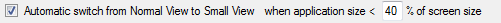In this case, when the total surface covered by the application is less than 40 % of the total screen surface, the application switches to Small View.

Both Normal View and Small View can be customised.

Normal ViewSmall View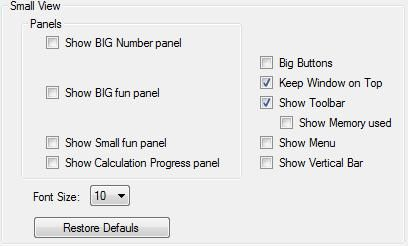In this example, if you resize your calculator window to a size less than 40 % of the surface area, the calculator's view will change from Normal View with buttons, captions, calculation numbers, bigger font to Small View, without buttons, smaller font, always-on-top, etc.

To use the Calculator without buttons, you'll need to use keyboard shortcuts for operations that are non-standard.

The Options window has the following button:Showing the following window: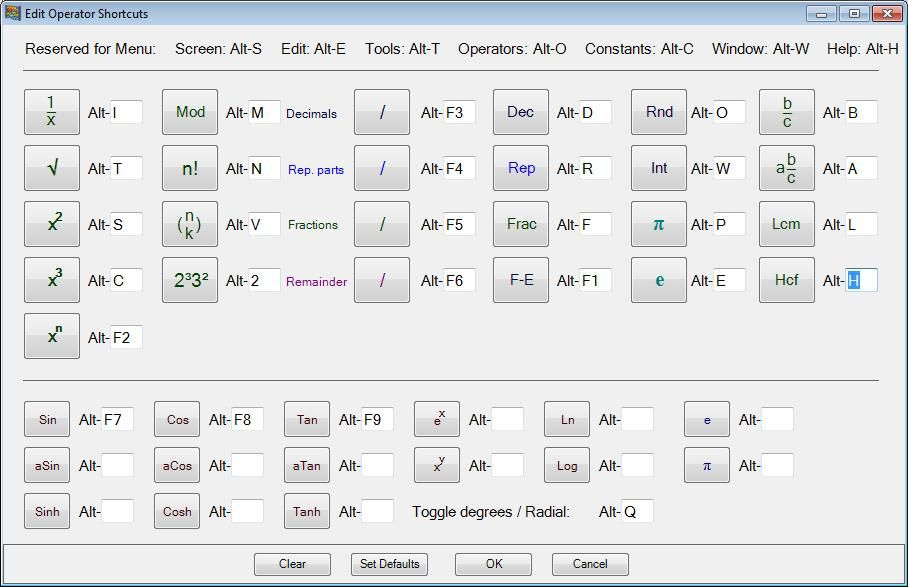Here you can define your own shortcuts.

## Export to the Clipboard as Image

When you want to display your calculations in another program, like Word, you can easily copy and export data from the calculator.

Via Edit - Copy last Line as Image to Clipboard and Copy Screen as Image to Clipboard, the calculation or screen can be copied as an image to the clipboard. This will result in an identical view as is shown within the calculator.

For example, add the fractions 1/2 and 1/3 = in the calculator, then copy line as image, paste it in Word, you will get: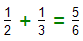This is the identical view as appeared on your calculator screen.

Export as .txt file

Via the button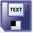Will the entire screen be copied to a .txt file. The same rules apply as under Export to Clipboard as text

Interested?